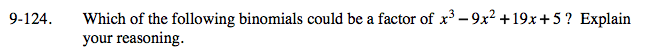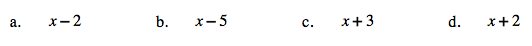Home > A2C > Chapter 9 > Lesson 9.3.1 > Problem9-124

9-124.
1. Which of the following binomials could be a factor of x3 − 9x2 + 19x + 5? Explain your reasoning. Homework Help ✎

1. x − 2

2. x − 5

3. x + 3

4. x + 2Binomial (b) because 5 is a factor of the last term, but 2 and 3 are not.